# Chapter 12 : Spectroscopic methods

Spectroscopic methods are destined to determine the composition of samples containing one or several species on the basis of their energy levels. We have seen that the electrons of atoms don’t have access to any value of energy. They are limited to a few values of energy, the energy orbital’s/levels 1s, 2s, 2p, etc, on which they are distributed following the rule of Fermi, beginning with the energy levels with lowest energies and filling the levels until there are no more electron to place.

The electrons of highest energy can be excited and reach orbitals of higher energies if they receive the exact amount of energy ΔE separating the two energy levels, in the form of heat of or photons. We say that the electron passes from its ground state to an excited state.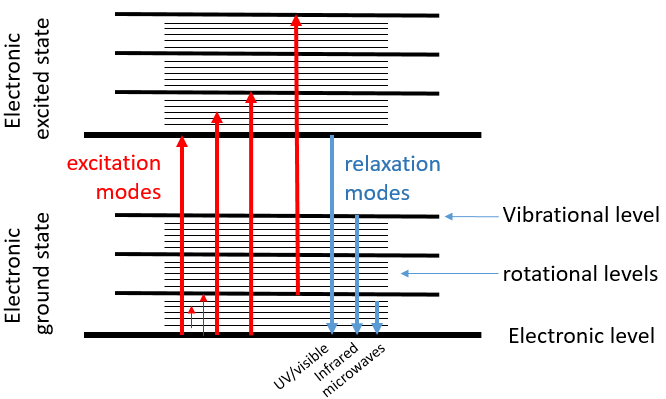When an excited electron goes back to its ground state, it generates a photon with the same energy ΔE=hν. The frequency of the photon is characteristic of the atom or of the molecule from which it is emitted. The frequency ν, as a unit of measure, is the amount of times that a process occurs over a period of one second. In the case of photons, it is the amount of waves that passes by a point over one second (units: hertz). The wavelength λ=c/ν is the length of one wave, or the distance between two summits, in meter. The wavenumber n is the reciprocal of the wavelength (1/λ, units: cm-1).  The difference between the frequency and the wavenumber is that wavenumber has nothing to do with the velocity of the wave. This makes it useful for situations where the velocity or frequency are not fixed.

For instance, when we heat up a metal, it changes of colour because the excited electrons emit photons within the range of the visible. The spectre of the visible is composed of all the colours that humans can see. Each colour corresponds to a photon of a given wavelength. The violet is the visible colour with the smallest wavelength (390nm) (or the largest wavenumber) and the red is the visible colour that has the largest wavelength (780nm) (or the smallest wavenumber). Ironically the colour that we see on a given material is not the colour that the material absorbs but all the other colours that haven’t been absorbed and that are thus reflected by the material towards our eye.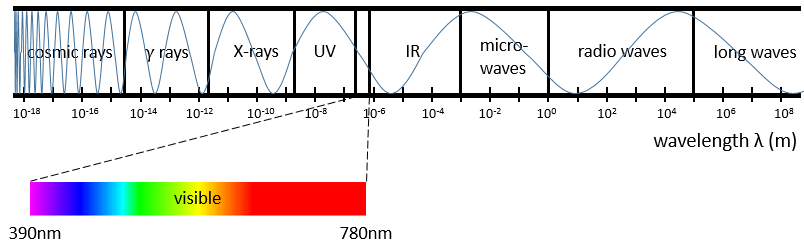Radiations are not limited to the visible spectre. There are photons with wavelength above 780nm and below 390nm. Radiations with a wavenumber smaller than the visible spectre are called infrared (the red being the colour with the smallest wavenumber). If we continue to decrease the wavenumber (or increase the wavelength) we reach the microwaves and then the radio waves. On the other side of the visible spectre we find the ultraviolet radiations (the violet having the largest wavenumber of the visible spectre), then the X-rays and finally the γ-rays. As the energy of a photon is E=hν, the photons gain energy when we go from the radio waves towards the gamma rays. X-rays have enough energy to extract a deep electron (not an electron of valence) from an atom. No need to say the damages that gammas rays from radioactive elements can do.

The electronic transitions we discussed previously correspond to radiations in the UV/visible spectre. Those transitions are not the only transitions that can take place. Between each electronic level we find vibration levels and between the vibration levels we find rotational levels. The transitions between vibration levels are in the range of the IR while rotational transitions are in the range of the microwaves. These transitions lead to an emission of heat and that’s exactly how the microwaves oven works: the food is bombarded by radiations in the range of microwaves and the molecules of the food rotate, generating the heating of the food.

Spectroscopy

The absorption of radiations of a solution depends thus on its composition. We can whether measure how a solution absorbs a given radiation or how this solution emits photons when its molecules are excited.

Absorption spectroscopy

The absorbance A of a solution is its capacity to block/absorb radiations. The absorbance A(λ) depends on the wavelength of the radiation.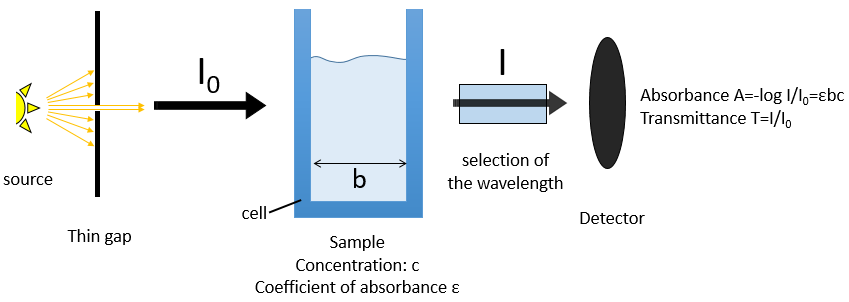If we consider an incoming ray with an intensity I0 that is heading towards a cell of length b containing a solution with a concentration c, the intensity I of the ray behind the cell is decreased by dI proportionally to the length of the cell (a longer cell absorbs more) and to the concentration of the solution (a more concentrated solution absorbs more).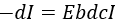E is the coefficient of absorbance of the solution that does not depends on the concentration nor on the size of the cell and dc is the concentration of solution through which the ray passed through, i.e. the difference of concentration between inside and outside the cell.  We can rewrite this expression as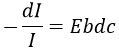and we can integer dI and dc between the emission of the ray and its detection, i.e. from I=I0 to I=I and from c=0 to c=c. It gives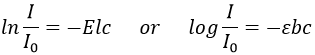The absorbance is thus given by the law of Beer-Lambert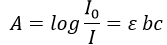We can also define the transmittance that is T=I/I0.

The absorbance is thus directly proportional to the concentration of the solution. Deviations from the linearity can be observed for concentrated solutions (c≠activity) or if some molecules of the sample are involved in an equilibrium.

The walls of the cell are made to let a maximum of the ray pass. They are usually in quartz (transparent between 200 and 380nm). A variation of the intensity of the ray can however be observed but can be determined using a white solution, i.e. a solution that only contain the solvent, with c=0 or a reference. The detector can be setup to define T=1 (or A=0) with the white solution. As the absorbance is directly proportional to the concentration of the solution we can determine the concentration of our sample if we measured the absorbance of our white solution and of an etalon solution the concentration of which is known.

If several species are in the solution, the absorbance is the sum of the individual absorbances.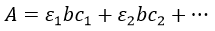To determine the concentrations, we need to repeat the experiment at several wavelengths. Don’t forget that epsilon depends on the wavelength.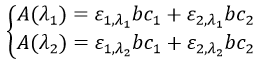We can thus determine c1 and c2 if the εi are known.

Experimental setup

The instrument is composed of 5 major parts:

• A stable source of radiant energy,
• A transparent device destined to contain the sample,
• A system to select a range of the spectre,
• A detector that can transform the luminous signal into an electric signal,
• A device to treat the signal.

The source of the ray has to be a monochromatic lamp, sending only photons with a given wavelength. It can be for instance a hollow cathode lamp or a laser source.  However, there is always a small range of wavelengths that is emitted. The impact can be limited if the wavelength is so that the ε coefficients don’t vary too much on this range of wavelengths.

The cell is usually in quartz and its size is usually 1cm long for spectroscopy of solutions. For gases the thickness is between 1 and 100mm but the ray can pass several times in the cell with the use of mirrors, giving optical paths up to 120m. It is also possible to allow a flow of gas.

The selection of the wavelength is done by a combination of prisms/mirrors and thin gaps. The prisms separate the wavelengths of the incident light and the gaps only allow a small range of wavelengths to pass toward the detector.

The detector can also introduce some error in the measure. The relative intensity of those errors is large when A→0 or when it A is large. Again, a good choice of wavelength limits the experimental errors.

Molecular spectroscopy

Another way to use the absorption properties of solutions is to sweep a range of wavelengths and to detect the absorbance. It requires a source of light able to change of wavelength. If there were only the electronic levels, we would obtain discrete rays corresponding to the exact energy of the transition between the ground state of the electron and one excited state. However, the rotational and vibrational sublevels transform the rays into bands. The bands are thinned if we perform the spectroscopy of gases. In the case of UV-visible absorption spectroscopy, this technique is mostly used quantitatively. For instance we can follow the evolution of a reaction through the spectre of absorbance. If the products and the reactants are absorbing at different wavelengths, the intensity of the peaks characteristic of the products will increase over time while the peaks of the reactants decrease in size.

Chromophore: it is an organic covalent unsaturated group that absorbs in the UV (ex: C=C, C=O, NO2) with electrons that can be delocalised. The photons excite electrons that jump between energy levels that are extended π orbitals, created by a series of alternating single and double bonds, often in aromatic systems.

Auxochrome: A saturated group with free electrons that modifies the intensity and the wavelength of the absorption when bound to a chromophore.

Bathochromic shift: displacement of the absorption towards larger wavelengths (displacement towards the red) due to a solvent effect or a substitution.

Hypsochromic shift: displacement of the absorption towards smaller wavelengths (displacement towards the blue) due to a solvent effect or a substitution.

Hyperchromicity: increase of the intensity of the absorption.

Hypochromicity: decrease of the intensity of the absorption.